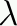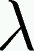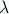# Perl contains the-calculus-Calculus (pronounced `lambda calculus') is a model of computation invented by Alonzo Church in 1934. It's analogous to Turing machines, but it's both simpler and more practical. Where the Turing machine is something like a model of assembly language, the-calculus is a model of function application. Like Turing machines, it defines a simplified programming language that you can write real programs in. Writing Turing machine programs is like writing in assembly language, but writing-calculus programs is more like writing in a higher-level language, because it has functions.

The two legal operations in the-calculus are to construct a function of one argument with a specified body, and to invoke one of these functions on an argument. What can be in the body of the function? Any legal expression, but expressions are limited to variables, function constructions, and function invocations. What can the argument be? It has to be another function; functions are all you have. With this tiny amount of machinery, we can construct a programming language that can express any computation that any other language can express.

Unlike most popular programming languages, Perl is powerful enough to express the-calculus directly, without the need to write a simulator. This means that if you want to try programming in the-calculus, you can do it directly in Perl, without having to implement a program to parse and evaluate-calculus expressions first. Perl's parser will parse-expresisons, if you write them properly, and its evaluator will evaluate them.

In the-calculus, a function with formal parameter x and body B is denotedx.B. In Perl, we write sub { my \$x = shift; B }.

To apply the function P to an argument Q, the usual-calculus notation is just (P Q). In Perl, we write \$P->(\$Q).

The only expressions not of either of these two forms are simple variables. To apply the functionv.B to some argument A is simple. The result is just B, but with any occurrences of v replaced with A instead. For example (x.(x (x y))(P Q)) reduces to ((P Q) ((P Q) y))---we replaced all the x's with (P Q)s.

By convention, function application is taken to be left-associative, so that (P Q R) is short for ((P Q) R).

From these few materials we can construct a programming system capable of performing arithmetic, constructing lists and trees, and expressing arbitrary recursive functions on these objects.

mjd-perl-memoize@plover.com